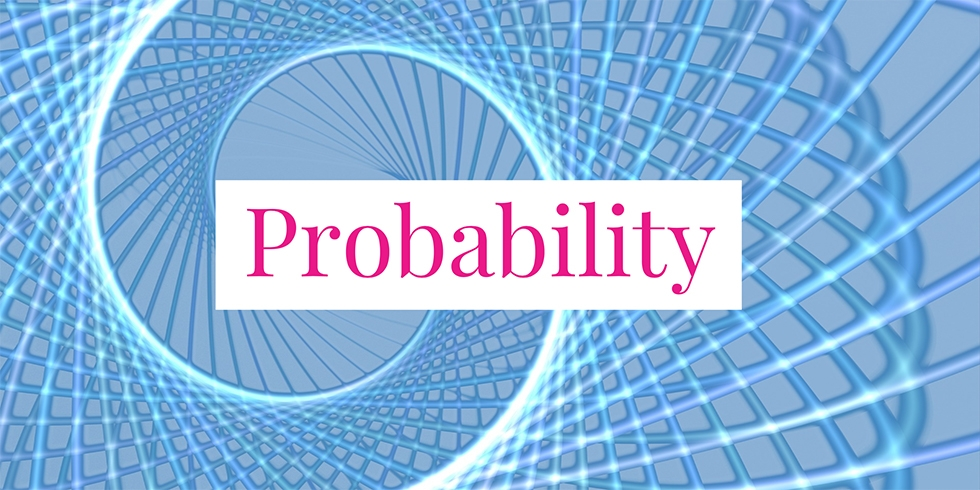# Find the Conditional Probability About Math Exam Experiment## Problem 740

A researcher conducted the following experiment. Students were grouped into two groups. The students in the first group had more than 6 hours of sleep and took a math exam. The students in the second group had less than 6 hours of sleep and took the same math exam.

The pass rate of the first group was twice as big as the second group. Suppose that $60\%$ of the students were in the first group. What is the probability that a randomly selected student belongs to the first group if the student passed the exam?Add to solve later

## Solution.

Let $E$ be the event that a student passes the exam.
Let $G_i$ be the event that a student belongs to the group $i$ for $i= 1, 2$. Then the desired probability is $P(G_1 \mid E)$. ($G_1$ is the first group and $G_2$ is the second group.)

Using these notation, the pass rate of the first group is expressed as $P(E \mid G_1)$. Similarly, the pass rate of the second group is $P(E \mid G_2)$. By assumption, the pass rate of the first group is twice as big as the second group. Hence, we have
$P(E \mid G_1) = 2\ P(E \mid G_2).$

The required probability that a randomly selected student belongs to the first group given that the student passes the exam is expressed as $P(G_1 \mid E)$.

Using the above equality and Bayes’ rule, we get
\begin{align*}
&P(G_1 \mid E)\\[6pt] &= \frac{P(G_1) P(E \mid G_1)}{P(E)} & \text{(by Bayes’ rule)}\\[6pt] &= \frac{P(G_1)P(E \mid G_1)}{P(E \mid G_1)P(G_1) + P(E \mid G_2)P(G_2)} & \text{(rule of total probability)}\\[6pt] &= \frac{P(G_1)\cdot 2P(E \mid G_2)}{2P(E \mid G_2)P(G_1) + P(E \mid G_2)P(G_2)} \\[6pt] &= \frac{P(G_1)\cdot 2}{2P(G_1) + P(G_2)}\\[6pt] &= \frac{0.6 \cdot 2}{2\cdot 0.6 + 0.4}\\[6pt] &= \frac{3}{4}.
\end{align*}

Therefore, the required probability is $P(G_1 \mid E) = \frac{3}{4}$.Add to solve later

### 1 Response

1.Jdm52 says:

I peruse this site mostly from my phone, so I don’t always have a pencil and paper with me. That being said, I used more less deduction to solve this problem which is more or less an intuitive method to employing the math.

What we care about is the relative proportions of students who passed the math exam, so the population constituting the first group represents 60% of the population leaving 40% for the other, or stated otherwise it is 1.5x larger. Then, that group also passed at 2x the rate, so in conjunction with increased population this means 3x (1.5*2) the amount of students from the first group passed meaning the ratio of students to pass from the first group to the second group is 3:1, thus the chance of selecting a student from the first group is 3/4.

The math in the solution is sound but I thought this comment might give an alternative illuminating intuitive rationale behind the math posted above.

This site uses Akismet to reduce spam. Learn how your comment data is processed.

###### More in Probability##### What is the Probability that Selected Coin was Two-Headed?

There are three coins in a box. The first coin is two-headed. The second one is a fair coin. The...

Close